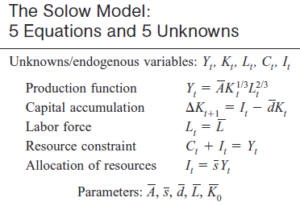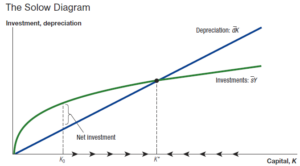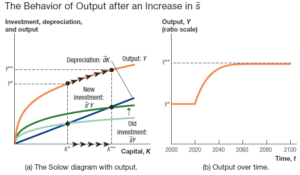Economics Tutors | Micro, Macro & Econometrics Tuitions | EconTutors

# Solow Growth Model – Macroeconomics Tutor in NYC and London

## Macroeconomics Tutor available for New York University (NYU), Columbia University, Yale, Princeton, UPenn, Brown, Duke, Cornell and Universities in London (LSE, Kings, UCL)

Our Macroeconomics tutor covers Solow Growth model in depth.These five key equations sum up macroeconomic relationships.

The capital accumulation equation summarizes the law of motion of capital. It states that capital next period is equal to capital this period, plus investment and less depreciation of capital.

The labor force is assumed to be constant such that Lt is equal to Lt+1, so there is no growth in population. Obviously, this is a simplified assumption and will be relaxed in a more detailed derivation.Initially, when the capital stock is at K0 the amount of investment per capita is higher than the amount of depreciation per capita. This creates net capital investment in the economy. Consequently, in the next period the amount of capital per capita increases, leading to higher output and higher investment. Note that investment per capita is savings rate times outper per capita. The steady state of capital is reached when the new investment in capital stock equals depreciation of capital stock. As a result, net change in capital is zero and the economy reaches a steady state of capital.Loading...Taking too long?Reload document
|Open in new tab

Get Started

## See the #1 economics mentoring platform in action

Request a demo